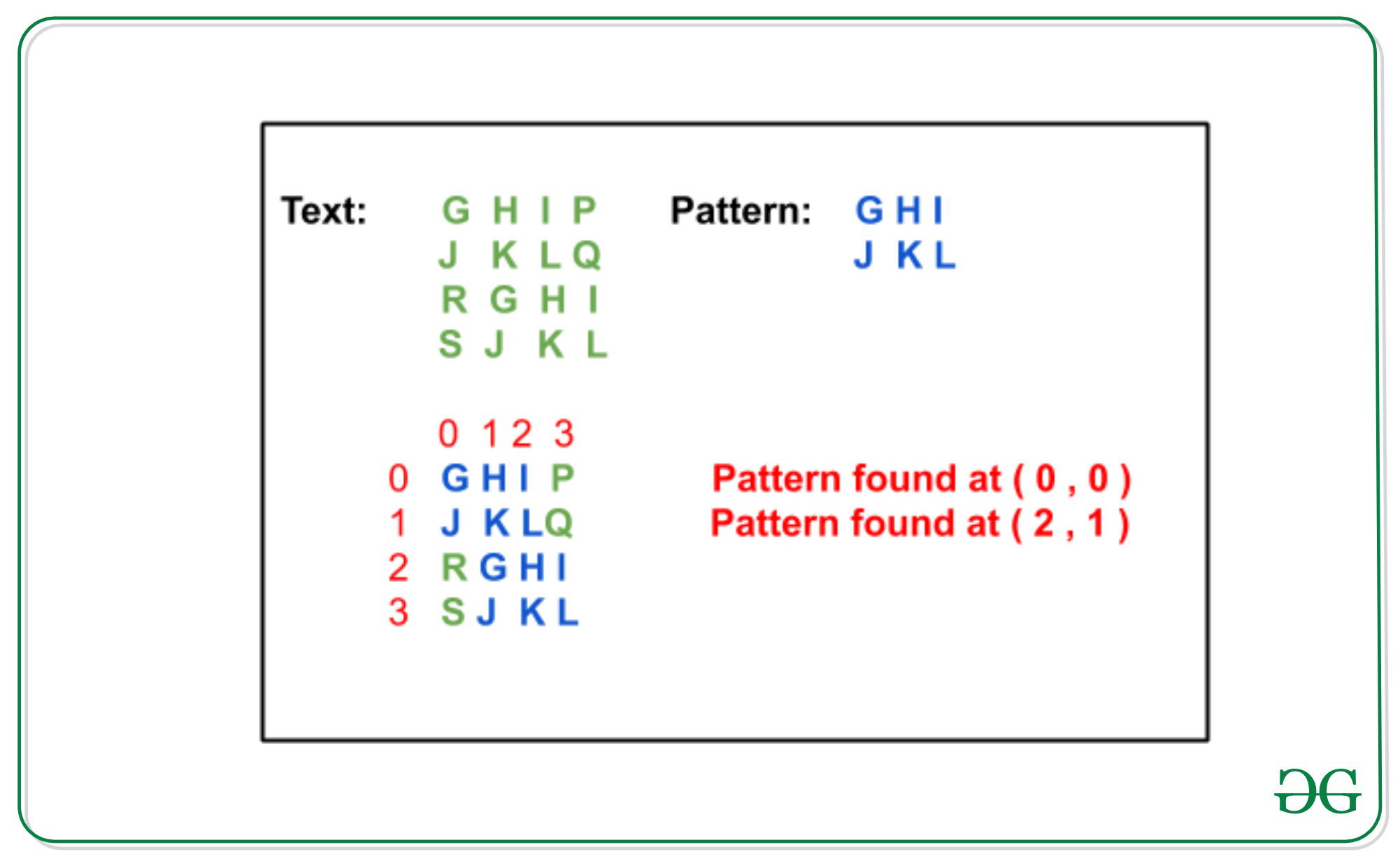Related Articles
Rabin-Karp algorithm for Pattern Searching in Matrix
• Difficulty Level : Hard
• Last Updated : 28 Jul, 2020

Given matrices txt[][] of dimensions m1 x m2 and pattern pat[][] of dimensions n1 x n2, the task is to check whether a pattern exists in the matrix or not and if yes then print the top mot indices od the pat[][] in txt[][]. It is assumed that m1, m2 ≥ n1, n2

Examples:

```Input:
txt[][] = {{G, H, I, P}
{J, K, L, Q}
{R, G, H, I}
{S, J, K, L}
}
pat[][] = {{G, H, I},
{J, K, L}
}
Output:
Pattern found at ( 0, 0 )
Pattern found at ( 2, 1 )
Explanation:Input:
txt[][] = { {A, B, C},
{D, E, F},
{G, H, I}
}
pat[][] = { {E, F},
{H, I}
}
Output:
Pattern found at (1, 1)
```

## Recommended: Please try your approach on {IDE} first, before moving on to the solution.

Approach: In order to find a pattern in a 2-D array using Rabin-Karp algorithm, consider an input matrix txt[m1][m2] and a pattern pat[n1][n2]. The idea is to find the hash of each columns of mat[][] and pat[][] and compare the hash values. For any column if hash values are equals than check for the corresponding rows values. Below are the steps:

1. Find the hash values of each column for the first N1 rows in both txt[][] and pat[][] matrix.
2. Apply Rabin-Karp Algorithm by finding hash values for the column hashes found in step 1.
3. If a match is found compare txt[][] and pat[][] matrices for the specific rows and columns.
4. Else slide down the column hashes by 1 row in the txt matrix using a rolling hash.
5. Repeat steps 2 to 4 for all the hash values and if we found any pat[][] match in txt[][] then print the indices of top most cell in the txt[][].

To find the hash value: In order to find the hash value of a substring of size N in a text using rolling hash follow below steps:

1. Remove the first character from the string: hash(txt[s:s+n-1])-(radix**(n-1)*txt[s])%prime_number
2. Add the next character to the string: hash(txt[s:s+n-1])*radix + txt[n]

Below is the implementation of the above approach:

## Python3

 `# Python implementation for the ``# pattern matching in 2-D matrix`` ` `# Function to find the hash-value ``# of the given columns of text``def` `findHash(arr, col, row):``    ``hashCol ``=` `[]``    ``add ``=` `0``    ``radix ``=` `256`` ` `    ``# For each column``    ``for` `i ``in` `range``(``0``, col):`` ` `        ``for` `j ``in` `reversed``(``range``(``0``, row)):``            ``add ``=` `add ``+` `(radix``*``*``(row``-``j``-``1``) ``*` `                         ``ord``(arr[j][i]))``%` `101``        ``hashCol.append(add ``%` `101``);``        ``add ``=` `0``    ``return` `hashCol`` ` `# Function to check equality of the ``# two strings``def` `checkEquality(txt, row, col, flag):``    ``txt ``=` `[txt[i][col:patCol ``+` `col] ``           ``for` `i ``in` `range``(row, patRow ``+` `row)]`` ` `# If pattern found``    ``if` `txt ``=``=` `pat:``        ``flag ``=` `1``        ``print``(``"Pattern found at"``, \``              ``"("``, row, ``", "``, col, ``")"``)``    ``return` `flag``     ` `# Function to find the hash value of``# of the next column using rolling-hash``# of the Rabin-karp``def` `colRollingHash(txtHash, nxtRow):`` ` `    ``radix ``=` `256`` ` `    ``# Find the hash of the matrix``    ``for` `j ``in` `range``(``len``(txtHash)):``        ``txtHash[j] ``=` `(txtHash[j]``*``radix \``                      ``+` `ord``(txt[nxtRow][j]))``%` `101``        ``txtHash[j] ``=` `txtHash[j] ``-` `(radix``*``*``(patRow) ``*` `                     ``ord``(txt[nxtRow``-``patRow][j]))``%` `101` `        ``txtHash[j] ``=` `txtHash[j]``%` `101``    ``return` `txtHash``     ` ` ` `# Function to match a pattern in ``# the given 2D Matrix``def` `search(txt, pat):``     ` `# List of the hashed value for``    ``# the text and pattern columns``    ``patHash ``=` `[]``    ``txtHash ``=` `[]`` ` `    ``# Hash value of the ``    ``# pat_hash and txt_hash``    ``patVal ``=` `0``    ``txtVal ``=` `0` ` ` `    ``# Radix value for the input characters``    ``radix ``=` `256``     ` `    ``# Variable to determine if``    ``# pattern was found or not``    ``flag ``=` `0``     ` `    ``# Function call to find the``    ``# hash value of columns``    ``txtHash ``=` `findHash(txt, txtCol, patRow)  ``    ``patHash ``=` `findHash(pat, patCol, patRow)``     ` `    ``# Calculate hash value for patHash``    ``for` `i ``in` `range``(``len``(patHash)):``        ``patVal ``=` `patVal \``                 ``+` `(radix``*``*``(``len``(patHash)``-``i``-``1``) ``*` `                 ``patHash[i]``%` `101``)``    ``patVal ``=` `patVal ``%` `101``     ` `     ` `    ``# Applying Rabin-Karp to compare``    ``# txtHash and patHash``    ``for` `i ``in` `range``(patRow``-``1``, txtRow):``        ``col ``=` `0``        ``txtVal ``=` `0``         ` `        ``# Find the hash value txtHash ``        ``for` `j ``in` `range``(``len``(patHash)):``            ``txtVal ``=` `txtVal\``                     ``+` `(radix``*``*``(``len``(patHash)``-``j``-``1``) ``*` `                     ``txtHash[j])``%` `101``        ``txtVal ``=` `txtVal ``%` `101``         ` `        ``if` `txtVal ``=``=` `patVal:``            ``flag ``=` `checkEquality(\``                     ``txt, i ``+` `1``-``patRow, col, flag)``             ` `        ``else``:`` ` `            ``# Roll the txt window by one character ``            ``for` `k ``in` `range``(``len``(patHash), ``len``(txtHash)):`` ` `                ``txtVal ``=` `txtVal \``                         ``*` `radix ``+` `(txtHash[k])``%` `101``                ``txtVal ``=` `txtVal \``                         ``-` `(radix``*``*``(``len``(patHash)) ``*``                         ``(txtHash[k``-``len``(patHash)]))``%` `101``                ``txtVal ``=` `txtVal ``%` `101``                ``col ``=` `col ``+` `1`` ` `                ``# Check if txtVal and patVal are equal``                ``if` `patVal ``=``=` `txtVal:``                    ``flag ``=` `checkEquality(\``                           ``txt, i ``+` `1``-``patRow, col, flag)   ``                ``else``:``                    ``continue``                 ` `        ``# To make sure i does not exceed txtRow``        ``if` `i ``+` `1``
Output:
```Pattern found at ( 1 ,  1 )
```